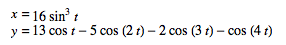Posted on February 14, 2015

# Animating a heart shaped curve with d3.js

Just something small for Valentines day. I found the formula for a heart shaped curve on the Wolfram website and wanted to animate the line that draws these points with d3.js.

I actually had in mind to make the line disappear again after a few seconds, so it would seem like a snake passing between the points, but I couldn’t find how to do this, so I just let the lines be. Now the end result reminds me of those spirographs from my childhood.

The formula for the heart curve, where `t` is in radians (I used a step size of 1), is'The mathematical formula for 'love''

Which translates to the following loop to create the data in JavaScript

``````var x, y;
var data = [];
for (var i = 0; i < 350; i++) {
t = i*0.1;
x = 16 * Math.pow(Math.sin(t),3);
y = 13 * Math.cos(t) - 5* Math.cos(2*t) - 2 * Math.cos(3*t) - Math.cos(4*t)
data[i] = [x,y];
}//for i
``````

If you make the steps for `t` small enough, you get an actual heart without the lines all over the place, but I quite liked the extra element. For completeness, here is what the line would look like with steps of `0.1`'Using a small enough step size creates a nice looking heart shape'

Here is the complete code for the animated line and for the one line. Happy Valentine’s Day!